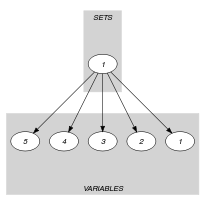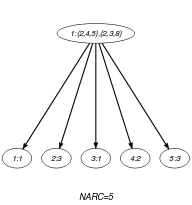## 5.333. roots

Origin
Constraint

$\mathrm{𝚛𝚘𝚘𝚝𝚜}\left(𝚂,𝚃,\mathrm{𝚅𝙰𝚁𝙸𝙰𝙱𝙻𝙴𝚂}\right)$

Arguments
 $𝚂$ $\mathrm{𝚜𝚟𝚊𝚛}$ $𝚃$ $\mathrm{𝚜𝚟𝚊𝚛}$ $\mathrm{𝚅𝙰𝚁𝙸𝙰𝙱𝙻𝙴𝚂}$ $\mathrm{𝚌𝚘𝚕𝚕𝚎𝚌𝚝𝚒𝚘𝚗}\left(\mathrm{𝚟𝚊𝚛}-\mathrm{𝚍𝚟𝚊𝚛}\right)$
Restrictions
 $𝚂\le |\mathrm{𝚅𝙰𝚁𝙸𝙰𝙱𝙻𝙴𝚂}|$ $\mathrm{𝚛𝚎𝚚𝚞𝚒𝚛𝚎𝚍}$$\left(\mathrm{𝚅𝙰𝚁𝙸𝙰𝙱𝙻𝙴𝚂},\mathrm{𝚟𝚊𝚛}\right)$
Purpose

$𝚂$ is the set of indices of the variables in the collection $\mathrm{𝚅𝙰𝚁𝙸𝙰𝙱𝙻𝙴𝚂}$ taking their values in $𝚃$; $𝚂=\left\{i|\mathrm{𝚅𝙰𝚁𝙸𝙰𝙱𝙻𝙴𝚂}\left[i\right].\mathrm{𝚟𝚊𝚛}\in 𝚃\right\}$. Positions are numbered from 1.

Example
$\left(\left\{2,4,5\right\},\left\{2,3,8\right\},〈1,3,1,2,3〉\right)$

The $\mathrm{𝚛𝚘𝚘𝚝𝚜}$ constraint holds since values 2 and 3 in $𝚃$ occur in the collection $〈1,3,1,2,3〉$ only at positions $𝚂=\left\{2,4,5\right\}$. The value $8\in 𝚃$ does not occur within the collection $〈1,3,1,2,3〉$.

Typical
 $|\mathrm{𝚅𝙰𝚁𝙸𝙰𝙱𝙻𝙴𝚂}|>1$ $\mathrm{𝚛𝚊𝚗𝚐𝚎}$$\left(\mathrm{𝚅𝙰𝚁𝙸𝙰𝙱𝙻𝙴𝚂}.\mathrm{𝚟𝚊𝚛}\right)>1$
Usage

Bessière et al. showed [BessiereHebrardHnichKiziltanWalsh05IJCAI] that many counting and occurence constraints can be specified with two global primitives: $\mathrm{𝚛𝚘𝚘𝚝𝚜}$ and $\mathrm{𝚛𝚊𝚗𝚐𝚎}$. For instance, the $\mathrm{𝚌𝚘𝚞𝚗𝚝}$ constraint can be decomposed into one $\mathrm{𝚛𝚘𝚘𝚝𝚜}$ constraint: $\mathrm{𝚌𝚘𝚞𝚗𝚝}$$\left(\mathrm{𝚅𝙰𝙻},\mathrm{𝚅𝙰𝚁𝚂},\mathrm{𝙾𝙿},\mathrm{𝙽𝚅𝙰𝚁}\right)$ iff $\mathrm{𝚛𝚘𝚘𝚝𝚜}$$\left(𝚂,\left\{\mathrm{𝚅𝙰𝙻}\right\},\mathrm{𝚅𝙰𝚁𝚂}\right)\wedge |𝚂|\mathrm{𝙾𝙿}\mathrm{𝙽𝚅𝙰𝚁}$.

$\mathrm{𝚛𝚘𝚘𝚝𝚜}$ does not count but collects the set of variables using particular values. It provides then a way of channeling. $\mathrm{𝚛𝚘𝚘𝚝𝚜}$ generalises, for instance, the $\mathrm{𝚕𝚒𝚗𝚔}_\mathrm{𝚜𝚎𝚝}_\mathrm{𝚝𝚘}_\mathrm{𝚋𝚘𝚘𝚕𝚎𝚊𝚗𝚜}$ constraint, $\mathrm{𝚕𝚒𝚗𝚔}_\mathrm{𝚜𝚎𝚝}_\mathrm{𝚝𝚘}_\mathrm{𝚋𝚘𝚘𝚕𝚎𝚊𝚗𝚜}$$\left(𝚂,\mathrm{𝙱𝙾𝙾𝙻𝙴𝙰𝙽𝚂}\right)$ iff $\mathrm{𝚛𝚘𝚘𝚝𝚜}$$\left(𝚂,\left\{1\right\},\mathrm{𝙱𝙾𝙾𝙻𝙴𝙰𝙽𝚂}.\mathrm{𝚋𝚘𝚘𝚕}\right)$, or may be used instead of the $\mathrm{𝚍𝚘𝚖𝚊𝚒𝚗}_\mathrm{𝚌𝚘𝚗𝚜𝚝𝚛𝚊𝚒𝚗𝚝}$.

Other examples of reformulations are given in [BessiereHebrardHnichKiziltanWalsh09].

Algorithm

In [BessiereHebrardHnichKiziltanWalsh06CP], Bessière et al. shows that enforcing hybrid-consistency on $\mathrm{𝚛𝚘𝚘𝚝𝚜}$ is NP-hard. They consider the decomposition of $\mathrm{𝚛𝚘𝚘𝚝𝚜}$ into a network of ternary constraints: $\forall i$, $i\in 𝚂⇒\mathrm{𝚅𝙰𝚁𝙸𝙰𝙱𝙻𝙴𝚂}\left[i\right].\mathrm{𝚟𝚊𝚛}\in 𝚃$ and $\mathrm{𝚅𝙰𝚁𝙸𝙰𝙱𝙻𝙴𝚂}\left[i\right].\mathrm{𝚟𝚊𝚛}⇒𝚃\wedge i\in 𝚂$. Enforcing bound consistency on the decomposition achieves bound consistency on $\mathrm{𝚛𝚘𝚘𝚝𝚜}$. Enforcing hybrid consistency on the decomposition achieves at least bound consistency on $\mathrm{𝚛𝚘𝚘𝚝𝚜}$, until hybrid consistency in some special cases:

• $\mathrm{𝑑𝑜𝑚}\left(\mathrm{𝚅𝙰𝚁𝙸𝙰𝙱𝙻𝙴𝚂}\left[i\right].\mathrm{𝚟𝚊𝚛}\right)\subset \underline{𝚃},\forall i\in \underline{𝚂}$,

• $\mathrm{𝑑𝑜𝑚}\left(\mathrm{𝚅𝙰𝚁𝙸𝙰𝙱𝙻𝙴𝚂}\left[i\right].\mathrm{𝚟𝚊𝚛}\right)\cap \overline{𝚃}=\varnothing ,\forall i\notin \overline{𝚂}$,

• $\mathrm{𝚅𝙰𝚁𝙸𝙰𝙱𝙻𝙴𝚂}$ are ground,

• $𝚃$ is ground.

Enforcing hybrid consistency on the decomposition can be done in $O\left(nd\right)$ with $n=|\mathrm{𝚅𝙰𝚁𝙸𝙰𝙱𝙻𝙴𝚂}|$ and $d$ the maximum domain size of $\mathrm{𝚅𝙰𝚁𝙸𝙰𝙱𝙻𝙴𝚂}\left[i\right].\mathrm{𝚟𝚊𝚛}$ and $𝚃$.

Systems

roots in Gecode, roots in MiniZinc.

related: $\mathrm{𝚊𝚖𝚘𝚗𝚐}$ (can be expressed with $\mathrm{𝚛𝚘𝚘𝚝𝚜}$), $\mathrm{𝚊𝚜𝚜𝚒𝚐𝚗}_\mathrm{𝚊𝚗𝚍}_\mathrm{𝚗𝚟𝚊𝚕𝚞𝚎𝚜}$ (can be expressed with $\mathrm{𝚛𝚘𝚘𝚝𝚜}$ and $\mathrm{𝚛𝚊𝚗𝚐𝚎}$), $\mathrm{𝚊𝚝𝚕𝚎𝚊𝚜𝚝}$, $\mathrm{𝚊𝚝𝚖𝚘𝚜𝚝}$ (can be expressed with $\mathrm{𝚛𝚘𝚘𝚝𝚜}$), $\mathrm{𝚌𝚘𝚖𝚖𝚘𝚗}$ (can be expressed with $\mathrm{𝚛𝚘𝚘𝚝𝚜}$ and $\mathrm{𝚛𝚊𝚗𝚐𝚎}$), $\mathrm{𝚌𝚘𝚞𝚗𝚝}$ (can be expressed with $\mathrm{𝚛𝚘𝚘𝚝𝚜}$), $\mathrm{𝚍𝚘𝚖𝚊𝚒𝚗}_\mathrm{𝚌𝚘𝚗𝚜𝚝𝚛𝚊𝚒𝚗𝚝}$, $\mathrm{𝚐𝚕𝚘𝚋𝚊𝚕}_\mathrm{𝚌𝚊𝚛𝚍𝚒𝚗𝚊𝚕𝚒𝚝𝚢}$, $\mathrm{𝚐𝚕𝚘𝚋𝚊𝚕}_\mathrm{𝚌𝚘𝚗𝚝𝚒𝚐𝚞𝚒𝚝𝚢}$ (can be expressed with $\mathrm{𝚛𝚘𝚘𝚝𝚜}$), $\mathrm{𝚜𝚢𝚖𝚖𝚎𝚝𝚛𝚒𝚌}_\mathrm{𝚊𝚕𝚕𝚍𝚒𝚏𝚏𝚎𝚛𝚎𝚗𝚝}$, $\mathrm{𝚞𝚜𝚎𝚜}$ (can be expressed with $\mathrm{𝚛𝚘𝚘𝚝𝚜}$ and $\mathrm{𝚛𝚊𝚗𝚐𝚎}$).

Keywords
Derived Collection
$\mathrm{𝚌𝚘𝚕}\left(\mathrm{𝚂𝙴𝚃𝚂}-\mathrm{𝚌𝚘𝚕𝚕𝚎𝚌𝚝𝚒𝚘𝚗}\left(𝚜-\mathrm{𝚜𝚟𝚊𝚛},𝚝-\mathrm{𝚜𝚟𝚊𝚛}\right),\left[\mathrm{𝚒𝚝𝚎𝚖}\left(𝚜-𝚂,𝚝-𝚃\right)\right]\right)$
Arc input(s)

$\mathrm{𝚂𝙴𝚃𝚂}$ $\mathrm{𝚅𝙰𝚁𝙸𝙰𝙱𝙻𝙴𝚂}$

Arc generator
$\mathrm{𝑃𝑅𝑂𝐷𝑈𝐶𝑇}$$↦\mathrm{𝚌𝚘𝚕𝚕𝚎𝚌𝚝𝚒𝚘𝚗}\left(\mathrm{𝚜𝚎𝚝𝚜},\mathrm{𝚟𝚊𝚛𝚒𝚊𝚋𝚕𝚎𝚜}\right)$

Arc arity
Arc constraint(s)
$\begin{array}{c}\mathrm{𝚒𝚗}_\mathrm{𝚜𝚎𝚝}\left(\mathrm{𝚟𝚊𝚛𝚒𝚊𝚋𝚕𝚎𝚜}.\mathrm{𝚔𝚎𝚢},\mathrm{𝚜𝚎𝚝𝚜}.𝚜\right)⇔\hfill \\ \mathrm{𝚒𝚗}_\mathrm{𝚜𝚎𝚝}\left(\mathrm{𝚟𝚊𝚛𝚒𝚊𝚋𝚕𝚎𝚜}.\mathrm{𝚟𝚊𝚛},\mathrm{𝚜𝚎𝚝𝚜}.𝚝\right)\hfill \end{array}$
Graph property(ies)
$\mathrm{𝐍𝐀𝐑𝐂}$$=|\mathrm{𝚅𝙰𝚁𝙸𝙰𝙱𝙻𝙴𝚂}|$

Graph model
##### Figure 5.333.1. Initial and final graph of the $\mathrm{𝚛𝚘𝚘𝚝𝚜}$ constraint(a) (b)